# Matrix

(redirected from Nail matrix)
Also found in: Dictionary, Thesaurus, Medical, Legal, Financial.
Related to Nail matrix: nail root

## matrix,

in mathematics, a rectangular array of elements (e.g., numbers) considered as a single entity. A matrix is distinguished by the number of rows and columns it contains. The matrix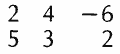is a 2×3 (read "2 by 3") matrix, because it contains 2 rows and 3 columns. A matrix having the same number of rows as columns is called a square matrix. The matrixis a 2×2 matrix, or square matrix of order 2; a square matrix of order n contains n rows and n columns. Definitions are made for certain operations with matrices; for example, a matrix may be multiplied by a number, and two matrices of the same order may be added or multiplied using an algebra of matrices that has been developed. Matrices find application in such fields as vector analysis and the solution of systems of linear equations by means of electronic computers.

### Bibliography

See R. C. Dorfi, Matrix Algebra (1969).

## Matrix

in cytology, the homogeneous fine-grained matter that fills intracellular structures (organoids) and the spaces between them.

There are three types of matrix: cytoplasmic matrix, which, depending on the physiological state of the cell, is capable of viscid flow or elastic deformation; mitochondrial matrix, the semifluid matter that fills the spaces between the cristae, or crests, of the mitochondria; and the matrix of the nucleus, plastids, and other organoids. Cytoplasmic matrix consists chiefly of protein molecules aggregated to various degrees and serving as a supportive medium for the cellular organoids. It holds basal bodies, centrioles, filaments, microtubules, and other fibrillar structures whose functions have not been completely elucidated.

### REFERENCE

Frey-Wyssling, A., and K. Mühlthaler. Ul’trastruktura rastitel’noi kletki. Moscow, 1968. (Translated from English.)
Loewy, A., and F. Siekevitz. Struktura i funktsii kletki. Moscow, 1971. (Translated from English.)

## Matrix

in mathematics, a system of elements aij (numbers, functions, or other quantities for which there are defined algebraic operations) arranged in the form of a rectangular array. If the array has m rows and n columns, we speak of an (m × n) matrix (m by n matrix). It is denoted byand in abbreviated notation, by ║aij║ or (aij). Both finite matrices and matrices with an infinite number of rows or columns are considered.

A matrix consisting of a single row is called a row matrix, and that consisting of a single column is called a column matrix. If m = n, the matrix is called a square matrix of order n. A square matrix in which only the diagonal elements α = αii are nonzero is called a diagonal matrix and is denoted by diag (α1, …, αn). If all αi = a, we speak of a scalar matrix. When α = 1, the matrix is called an identity matrix and is denoted by E. A matrix all of whose elements are zero is called a zero matrix.

By interchanging the rows and columns in a given matrix A, we obtain the transpose A′, or AT, of A. If we replace the elements of a matrix by their complex conjugates, we obtain the complex conjugate matrix Ā of A. If we replace the elements of the transpose A′ of Ā by their complex conjugates, we obtain the matrix A*, called the conjugate transpose of A. The determinant of a square matrix A is denoted by │A│, or det A. A determinant of kth order consisting of elements at the intersection of some k rows and k columns of the matrix A in their natural arrangement is called a minor of kth order of the matrix. The rank of a matrix is the maximal order of the nonzero minors of the matrix.

Operations on matrices. The product of a rectangular (m × n) matrix A and the number α is the matrix whose elements are obtained by multiplying every element aij of A by α: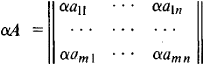Addition is defined for identically structured rectangular matrices, and the elements of the sum are the sums of the corresponding elements of the two summands, that is,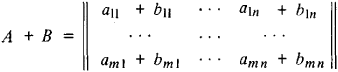Multiplication of matrices is defined only for rectangular matrices in which the number of columns of the first factor is equal to the number of rows of the second. The product of the (m × p) matrix A and the (p × n) matrix B is the (m × n) matrix C with elements

cij = ai1b1j + ai2b2j + … + aipbpj

i = 1, …, m j = 1, …, n

These three operations on matrices possess properties similar to those of the operations on numbers. The exception is the noncommutativity of matrix multiplication, which means that the equality AB = BA may not hold. If AB = BA, then the matrices A and B are said to commute. Moreover, the product of two matrices may be the zero matrix even though neither factor is zero. The following rules hold:The determinant of the product of two square matrices is equal to the product of their determinants.

It is often convenient to divide a matrix into blocks, each of which constitutes a matrix of lesser dimension, by drawing a line through the entire matrix from left to right or from top to bottom. When multiplying such a block matrix by a number, it is necessary to multiply each block by that same number. The addition and multiplication of matched block matrices are carried out as if the blocks were actully numbers.

The square matrix A = (aij) is nonsingular if its determinant is not zero; otherwise the matrix is singular. A matrix A−1 is an inverse of the square matrix A if AA−1 = E in this case, ajk(−1) = Aki/│A│, where Aki denotes the cofactor of aki,. The nonsingularity of a matrix A is a necessary and sufficient condition for the existence of an inverse of A. If A has an inverse, then that inverse is necessarily unique and commutes with A. We have

(AB)−1 = B−1A−1

Of great interest is the generalized inverse (or pseudoinverse) matrix A+, defined both for any rectangular matrix and for a singular square matrix. This matrix is defined by the four equalities

AA+A = A A+AA+ = A

AA+ = (AA+)* A+A = (A+A)*

Square matrices. The nth power An of the matrix A is the product of n factors equal to A. An expression of the form α0An + α1An−1 + … + αnE, where α0, α1, …, αn are numbers, is called the value of the polynomial α0tn + α1tn−1 + … + αnE at (the square matrix) A. The rules of operation on polynomials in a given matrix A are identical to the rules of operation on algebraic polynomials. We can also consider analytic functions of a matrix. In particular, if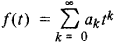is a series converging in the entire complex plane (for example, if f(t) = et) then the infinite series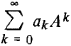is a convergent series for any matrix A and it is natural to put its sum equal to f(A). But if f(t) has a finite circle of convergence, then f(A) is defined by this series for “sufficiently small” matrices.

Analytic functions of a matrix play a major role in the theory of differential equations. Thus, a system of ordinary differential equations with constant coefficients, written in matrix notation in the form

dx/dt = AX

(here, X is the column of unknown functions), has the solution x = eAtC, where C is a column of arbitrary constants.

A nonzero column vector X such that AX = λX is called an eigenvector of the matrix A. In this equality, the coefficient λ can only be one of the roots of the polynomial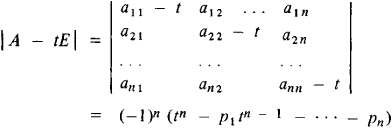which is called the characteristic polynomial of the matrix A. These roots are called the eigenvalues, or characteristic numbers, of A. The coefficients of the characteristic polynomial can be expressed in terms of sums of certain minors of A. In particular, p1 = a11 + • • • + a1n = Tr A (trace of A) and pn = (−1)n−1A│ We have the Cay ley-Hamilton theorem: if 0(t) is the characteristic polynomial of the matrix A, then 0(A) = 0, so that A is a “root” of its characteristic polynomial.

A matrix A is said to be similar to a matrix B if there exists a nonsingular matrix C such that B = C−1AC. It can be easily verified that similar matrices have identical characteristic polynomials.

Matrix calculus. Matrices are useful tools for investigating many problems in theoretical and applied mathematics. One of the most important problems is that of finding solutions of systems of linear algebraic equations. In matrix notation these equations are written in the form

AX = F

where A is the coefficient matrix; X is the desired solution, written in the form of a column of n elements; and F is the column of free terms consisting of m elements. If A is a square nonsingular matrix, then the system has the unique solution X = A−1F. If A is a rectangular (m × n) matrix of rank k, then the solution may not exist or may not be unique. When no solution exists, a generalized solution giving a minimum of the sum of the squares of the discrepancies may prove useful. When neither the exact nor generalized solution is unique, a normal solution, that is, a solution with the least sum of the squares of the components, is selected. The normal generalized solution is found from the formula X = A+F. The most important case is that of an overdetermined system k = n < m. In that case, the generalized solution is unique. When k = m < n (underdetermined system), then there exist infinitely many exact solutions and the formula above yields the normal solution.

No less important for many applications (in the theory of differential equations, the theory of small vibrations, quantum mechanics) is the solution of the complete or partial eigenvalue problem, which consists in finding all or some of the eigenvalues of a matrix and the corresponding eigenvectors or root vectors (certain generalized eigenvectors). This problem is closely related to the generalized eigenvalue problem, in which numbers and vectors are found such that AX = λBX (A and B are given matrices), and to many related problems.

The problem of reducing a square matrix to canonical form by means of similarity transformations is also directly connected with the complete eigenvalue problem. This form will be diag (λ1, …, λn,) if the matrix has n different eigenvalues λ1, …, λn,, or a Jordan form in the general case. (The Jordan canonical form of a square matrix is a matrix whose principal diagonal consists of the eigenvalues of the original matrix and whose diagonal just above or just below the principal diagonal consists of ones and zeros. The remaining entries are all equal to zero.)

Because of the great practical importance of these problems, there exist many different methods for their numerical solution. In addition to finding a numerical solution, it is also important to evaluate the “quality” of the solution found and to investigate the stability of the problem to be solved.

Special types of matrices. There exist numerous types of matrices, depending on the relationships between the elements. Some types arise naturally in various applications. Table 1 gives some of the most important types of square matrices.

Table 1. Types of matrices
MatrixDefining condition
SymmetricA = A′
Skew symmetricA = −A′
OrthogonalAA′ = E or A−1 = A′
Stochastic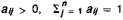HermitianA = A*
UnitaryAA* = E or A−1 = A*

We also note quasidiagonal matrices, that is, matrices whose nonzero elements are on the principal diagonal and on the diagonals on either side of the principal diagonal, for example, 2-diagonal and 3-diagonal matrices.

No less important are special types of matrices that are used as auxiliary matrices. These are the elementary matrices, which differ from the identity matrix in a single element, and matrices of rotation and reflection.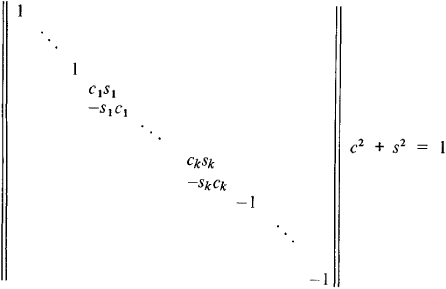Some additional types of matrices are unitary analogs of rotation and reflection matrices; upper (lower) triangular matrices, in which the elements below (above) the principal diagonal are equal to zero; and upper (lower) almost-triangular matrices (Hessenberg-type matrices), in which the elements below (above) the next diagonal below (above) the principal diagonal are equal to zero.

Matrix transformation. Numerical methods of solving systems of linear equations are usually based on the transformation of the systems by a series of multiplications on the left by suitable auxiliary matrices in order to obtain an easily solvable system. Elementary matrices, matrices of rotation, or matrices of reflection are used as auxiliary matrices for real matrices. A system with a nonsingular matrix reduces either to a system with a triangular matrix or to one with an orthogonal matrix. Theoretically this is equivalent to the representation of the coefficient matrix in the form of a product of two triangular matrices (under certain additional conditions) or in the form of a product of a triangular matrix and an orthogonal matrix in either order.

For an overdetermined system it is possible to arrive at a system with a triangular matrix of order n, whose solution yields a generalized solution of the initial system, by multiplying on the left by a chain of matrices of rotation or reflection.

To solve an eigenvalue problem, it is a good idea first to reduce a general matrix by means of a similarity transformation to a Hessenberg-type matrix (in the case of a symmetric matrix to a 3-diagonal matrix) and then use the most effective iteration methods. Such a preliminary reduction can be attained by means of a chain of similarity transformations involving elementary matrices, matrices of rotation, or matrices of reflection.

History. The concept of matrix was introduced by W. Hamilton and A. Cayley in the mid-19th century. The foundations of this theory were created by K. Weierstrass and F. Frobenius (second half of the 19th century and early 20th). I. A. Lappo Danilevskii developed the theory of analytic functions of several matrix arguments and applied it to the study of systems of differential equations with analytic coefficients. Matrix notation has become widespread in modern mathematics and its applications. The matrix calculus is developing toward constructing effective algorithms for the numerical solutions of fundamental problems.

### REFERENCES

Smirnov V. I. Kurs vysshei matematiki, 9th ed., vol. 3, part 1. Moscow, 1967.
Mal’tsev, A. I. Osnovy lineinoi algebry, 3rd ed. Moscow, 1970.
Gantmakher, F. R. Teoriia matrits, 3rd ed. Moscow, 1967.
Wilkinson, J. H. Algebraicheskaia problema sobstvennykh znacheniL Moscow, 1970. (Translated from English.)
Faddeev, D. K., and V. N. Faddeeva. Vychislitel’nye melody lineinoi algebry, 2nd ed. Moscow-Leningrad, 1963.
Voevodin, V. V. Chislennye metody algebry: Teoriia i algorifmy. Moscow, 1966.
Lappo-Danilevskii, I. A. Primenenie funklsii ol matrits k leorii lineinykh sislem obyknovennykh differential’nykh uravnenii. Moscow, 1957.
Frazer, R. A., W. Duncan, and A. Collar. Teoriia matrits i ee prilozheniia k differential’nym uravneniiam i dinamike. Moscow, 1950. (Translated from English.)
Wasow, W., and G. Forsyth. Raznostnye metody resheniia differentsial’nykh uravnenii v chastnykh proizvodnykh. (Translated from English.) Moscow, 1963.

V. N. FADDEEVA

## Matrix

in printing:

(1) An interchangeable element of a casting mold with a recessed (sometimes photographic) image of a letter or symbol used in the casting of characters or lines. A matrix is a metal bar with the face of a letter or sign stamped (by pressure of a punch) or engraved on one of its edges. Characters or lines with a raised printing surface are formed on a matrix pressed to the mold by filling the cavities of the casting mold and face with liquid alloy. A distinction is made between typecasting, Linotype, and Monotype matrices, depending on the type of machine used to cast letters or lines.

A typecasting matrix is a steel bar of rectilinear cross section and a recessed image of a single letter or symbol. A set of typecasting matrices makes it possible to cast on a typecasting machine all letters of a single typeface used for manual composing.

In a Linotype machine a matrix line, which is mounted in front of the slit of a casting mold, is composed from individual matrices stored in the magazine. After the mold is filled with alloy, a solid metal line of type is formed.

In a Monotype machine a set of matrices is collected in a matrix frame. During casting, the required matrix is fitted over the slit of a casting mold. A line of type on a Monotype machine, unlike that on a Linotype machine, is formed from separate letters. The Monotype matrix has an aperture for threading onto the pivot of the matrix frame and a conical depression for precise fixing and pressing of the matrix to the casting mold.

In phototypesetting machines, matrices are used in which recessed images of characters are replaced by photographic images.

(2) The recessed imprint from a raised printing plate on plastic material (cardboard, plastic, and so on), used to produce stereo-type copies of the plate.

G. S. ERSHOV

## matrix

[′mā·triks]
(analytical chemistry)
The analyte as considered in terms of its being an assemblage of constituents, each with its own properties.
(computer science)
A latticework of input and output leads with logic elements connected at some of their intersections.
(electronics)
The section of a color television transmitter that transforms the red, green, and blue camera signals into color-difference signals and combines them with the chrominance subcarrier. Also known as color coder; color encoder; encoder.
The section of a color television receiver that transforms the color-difference signals into the red, green, and blue signals needed to drive the color picture tube. Also known as color decoder; decoder.
(engineering)
A recessed mold in which something is formed or cast.
(graphic arts)
In a type-casting machine, the portion of the mold that forms the letter face.
A heavy, unsized, unfinished paper that is used for molds for stereotype plates.
A master negative from which characters are projected in a photocomposition process.
(histology)
The intercellular substance of a tissue. Also known as ground substance.
The epithelial tissue from which a toenail or fingernail develops.
(materials)
A binding agent used to make an agglomerate mass.
(mathematics)
A rectangular array of numbers or scalars from a vector space.
(metallurgy)
The principal component of an alloy.
The precisely shaped form used as the cathode in electroforming.
(mycology)
The substrate on or in which fungus grows.
(petrology)
The continuous, fine-grained material in which large grains of a sediment or sedimentary rock are embedded. Also known as groundmass.

## matrix

1. In mortar, the cement paste in which the fine aggregate particles are embedded.
2. In concrete, the mortar in which the coarse aggregate particles are embedded.

## matrix

1. Anatomy the thick tissue at the base of a nail from which a fingernail or toenail develops
2. the intercellular substance of bone, cartilage, connective tissue, etc.
3.
a. the rock material in which fossils, pebbles, etc., are embedded
b. the material in which a mineral is embedded; gangue
4. (formerly) a mould used in the production of gramophone records. It is obtained by electrodeposition onto the master
5. a bed of perforated material placed beneath a workpiece in a press or stamping machine against which the punch operates
6. Metallurgy
a. the shaped cathode used in electroforming
b. the metal constituting the major part of an alloy
c. the soft metal in a plain bearing in which the hard particles of surface metal are embedded
7. the main component of a composite material, such as the plastic in a fibre-reinforced plastic
8. Maths a rectangular array of elements set out in rows and columns, used to facilitate the solution of problems, such as the transformation of coordinates. Usually indicated by parentheses: (
 abc def
)
9. Computing a rectangular array of circuit elements usually used to generate one set of signals from another
10. Obsolete the womb

## Matrix

[FidoNet] 1. What the Opus BBS software and sysops call FidoNet.

2. Fanciful term for a cyberspace expected to emerge from current networking experiments (see network, the).

3. The totality of present-day computer networks.

## matrix

An array of elements in row and column form. See x-y matrix.
References in periodicals archive ?
Nail matrix phenolization for treatment of ingrowing nail: technique report and recurrence rate of 267 surgeries.
The distal portion of the nail matrix is visible through the transparent nail plate as a white distally convex half moon, the lunula.
Another study by Grover C et al in 2015, using 10% NaOH to destroy the nail matrix instead of phenol, has found to have a better outcome with a mean duration of postoperative pain of 7.
4,18 The resultant pathologies were lacerations, tendon/nerve injuries, fractures, nail bed trauma and amputations of the nail matrix resulting into double nail deformity.
Supposedly, virus replication damages nail matrix and results in temporary nail dystrophy.
Horizontal grooves are formed proximally due to nail matrix damage and subsequently move distally with fingernail growth.
Many times such a nevus fades as the child ages, not because it regresses but because the nail matrix nevus cells reduce the production of melanin.
Over the years, lateral shoe pressure can lead to a change in the orientation of the nail matrix, as well as changing the thickness of the nail plate.
has rolled out Ardell nail mask, a brush-on/peel-off treatment to condition, nourish and revitalize the cuticle and nail matrix.
As a result of nail matrix function arrest, there is transverse ridging (beau's lines), temporary loss of nail plate.
Onychomycosis is a persistent nail infection of the nail bed, the nail matrix and or the nail plate, statistically it is the most common nail disorder in adults affecting up to 50% of patients presenting with nail disorders (Zaias et al 1996, Schlefman 1999, Ghannoum et al 2000).
and grooves are due to the nail matrix involvement, whereas onycholysis, subungual hyperkeratosis and discoloration attributable to the nail bed affection.

Site: Follow: Share:
Open / Close